# SSAT Elementary Level Math : How to find the area of a triangle

## Example Questions

← Previous 1 3

### Example Question #1 : Triangles

The altitude of a triangle is given as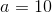, and its base as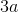. What is the area of the triangle?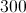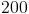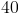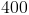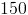Explanation:

The area of a triangle is given by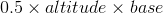.

altitude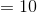base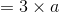=Therefore:

Area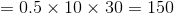### Example Question #1 : How To Find The Area Of A Triangle

What is the area of a triangle with a base of 11 and a height of 4?

14

60

6

44

22

22

Explanation:

The formula to find the area of a triangle is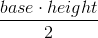. First, we should multiply 11 (base) x 4 (height), to get a total of 44. Next, we need to divide 44 by 2, which gives us a total area of 22.

### Example Question #1 : How To Find The Area Of A Triangle

You can find the area of a triangle if you know ___________.

the height and base

its angles

two side lengths

the perimeter

the height and base

Explanation: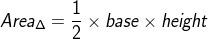### Example Question #4 : Triangles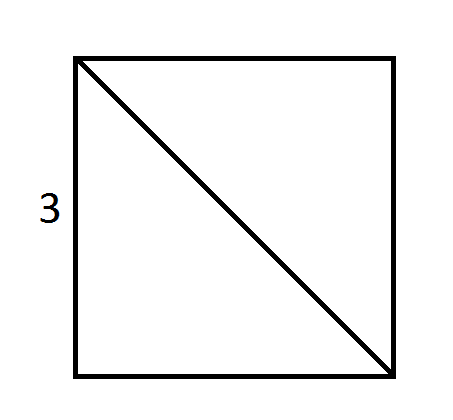The square shown above has a side length of 3 and is divided into two triangles by its diagonal. What is the area of one of the triangles?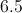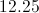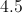Explanation:

The area of the square is side times side,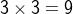.

Each triangle is half of the square,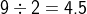.

### Example Question #1 : How To Find The Area Of A Triangle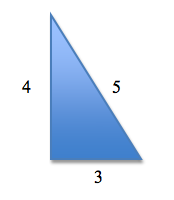What is the area of the triangle?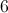Explanation:

The formula to find the area of a triangle is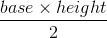First, we should multiply(base)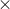(height), to get a total of.

Next, we need to divideby, which gives us a total area of### Example Question #2 : Triangles

An isosceles triangle has a base of 12 cm and a height of 6 cm. What is the area of the triangle?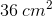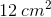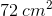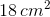Explanation:

To find the area of a triangle, you must multiplyby the base (12 cm) by the height (6 cm):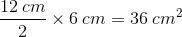Therefore the area of this triangle is.

### Example Question #592 : Plane Geometry

A triangle has a base of 14 and a height of 8. What is the area of the triangle?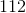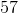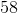Explanation:

To find the area of a triangle, multiply the base (14) by the height (8) and divide by 2: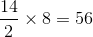Therefore the area of this triangle is.

### Example Question #1 : How To Find The Area Of A Triangle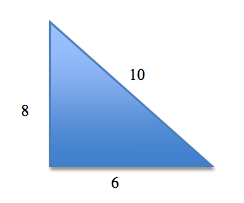What is the area of the triangle?

30

60

28

48

24

24

Explanation:

The formula to find the area of a triangle is.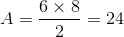### Example Question #3 : How To Find The Area Of A Triangle

If a triangle has a base of 3 inches and a height of 8 inches, what is the area of the triangle?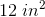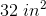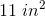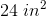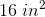Explanation:

The formula for the area of a triangle is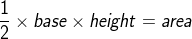.

Plug in the values given to solve the equation: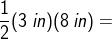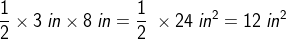### Example Question #1 : How To Find The Area Of A Triangle

A triangle has a base of 10 centimeters and a height of 12 centimeters. What is the area of the triangle?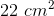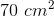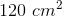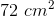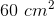Explanation:

The formula for the area of a triangle is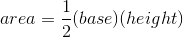.

Plug in the given values to solve for the area: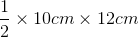=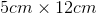=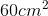The area of this triangle is.

← Previous 1 3

### All SSAT Elementary Level Math Resources